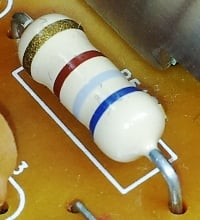# Resistor Guide

Welcome to the resistor guide!

The resistor guide will guide you in the world of resistors. This site is designed as an educational reference, serving as a reliable source for all information related to resistors. Several subjects will be covered including:

## Resistor fundamentalsWhen you just start working with resistors you might wonder, what is a resistor? or what exactly is the resistance of a resistor? Whenever you are working on circuits containing resistors, ohm's law describes the fundamental behavior of resistors. Kirchhoff's law can be used to analyse networks of resistors.

Resistors have several properties besides their rated resistance, such as their temperature coefficient, resistor noise and power rating. These resistor properties can be important to take into account depending on the application.

## Resistor standards and codes

Resistors are used and manufactured by thousands of companies and people worldwide. Therefore several standards exist which help to harmonize resistors throughout the world.

To identify the value of axial resistors, a resistor color code is used. This color code consists of several colored bands. Surface mount resistors are identified by a numerical resistor code. The nominal values of resistors are also standardized. Several ranges of preferred resistor values are available. Another important aspect of resistor standardization is the use of standardized resistor symbols. The IEC standard symbol of a fixed value resistor is shown.

## Resistor typesFixed resistor symbol

Several different types of resistor exist. The most used resistors are fixed value resistors, but also variable resistors are very common. The most used variable resistors are the potentiometer and rheostat. On the other hand there are a lot of resistors which have a variable resistance which is dependent on external factors such as temperature (thermistor), light (photoresistor), voltage (varistor) or magnetic fields (magneto resistor).

## Resistor materials

Resistors can be produced in several ways based on different materials. These different materials all have their own characteristics. One of the most used resistors nowadays is the carbon film resistor, other material resistors described on the resistor guide include:  carbon composition resistors, metal film resistors, metal oxide film resistors, foil resistors and wire-wound resistors.

## Resistor applications

Resistors are used in numerous applications, including virtually every circuit and electric device. In a lot of circuits they are often used, connected in series or in parallel. A frequent application in hobby projects is to use a resistor for a LED, to limit the current that will flow through the LED. A resistor can also be used to measure the current in a circuit, this is done using a shunt resistor.

Get Started

Get Started

Get Started
SHARE